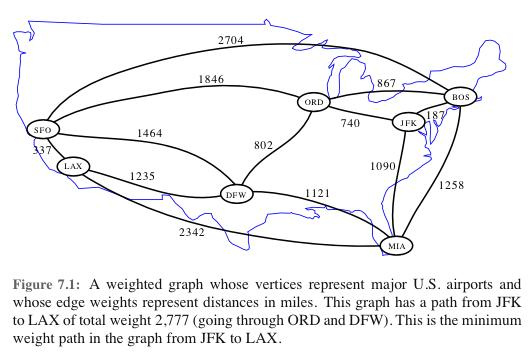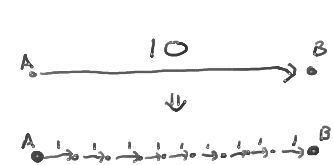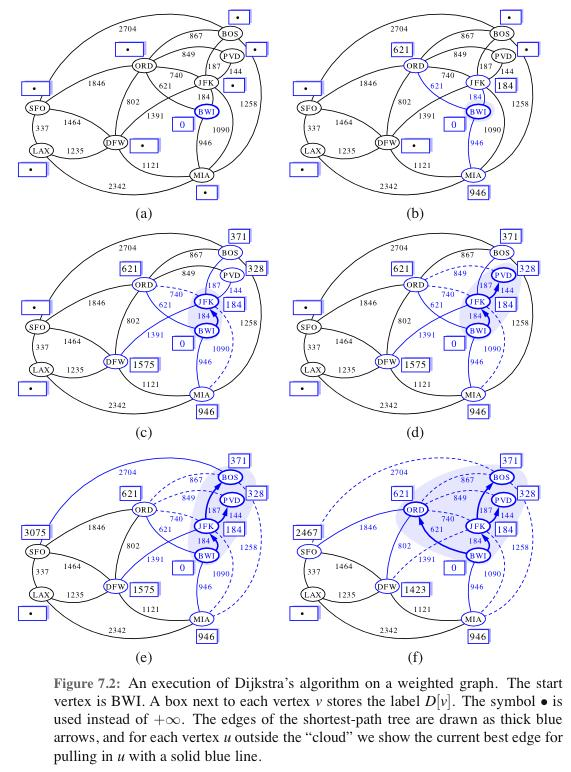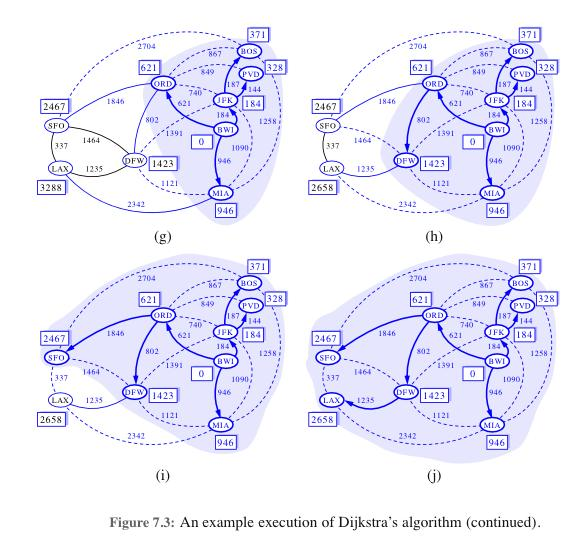# ShortestPathsByDijkstras

ClassS04CS141 | recent changes | Preferences

### Shortest Paths

Given a directed or undirected graph G=(V,E), with numeric weights on the edges, the length of a path P is defined to be the sum of the weights of the edges on the path.

The distance between two vertices u and w is defined to be the minimum length of any path from u to w.

The single-source shortest paths problem is, given the graph G and a start vertex s, to find distance(s,w) for all vertices w.

#### Example:?

### Dijkstra's algorithm

One way to solve this problem is to adapt breadth-first search to handle graphs with edge weights.

Imagine replacing each edge by multiple edges (in series). If the weight of an edge (u,w) is, say, W(u,v), then the edge will be replaced by a path consisting of W(u,v) unweighted edges:In the modified graph, the distances are the same is in the original graph. So, computing distances on the modified graph will give us the distances in the original graph.

This gives us an algorithm:

``` 1. Modify the graph by replacing each edge (u,v) by a path consisting of W(u,v) edges.
2. Run BFS on the modified graph.
3. For the vertices in the original graph, return the distances found by DFS in the modified graph.
```

The problem with this algorithm is that it can be slow. The running time is linear in the size of the modified graph. This size is (u,v)∈ E W(u,v), which can be quite large.

Instead, we can simulate the same process more efficiently. Consider BFS in this graph, as it starts. At any time, the set of visited vertices will be those that whose distances are at most some radius R from s:

We simulate the process by expanding the radius in larger steps, according to when it will next reach a vertex in the original graph:Here is pseudo-code:

``` 1.  PriorityQueue? Q;  HashTable distance<int,int>;
2.  Insert start vertex S into Q with key 0
3.  while (Q is not empty)
4.     Take the vertex v within minimum key k off the queue.
5.     distance[v] = k
6.     for each neighbor w of v do:
7.         if (! distance.exists[w])
8.            put w in Q with key distance[v] + WT(v,w)
9.            (or, if w is already in the Q, decrease the key to this if the current key is larger)
10. return distance[]
```

Is this algorithm correct? One argument that it is is that it simulates BFS on the modified graph, so the distances it discovers correspond to distances in the modified graph. Since distances in the modified graph are the same as in the original graph, the distances it discovers are accurate for the original graph.

Let's try a more direct argument. We will prove by induction as the algorithm proceeds that the distances that are set are correct.

base case: the first vertex removed from the queue is the start vertex s. it's distance is set to 0, which is correct.

inductive step:

In the general case, we have some set S of vertices whose distances are set already, and then in line 4 and 5 we take the vertex v in the queue with the minimum key k, and we set distance[v] = k. We have to argue that this is the correct setting. That is, that k is the length of the shortest path from s to v.

Let w be the vertex in S whose removal from the queue caused the key of v to be set to its current value. That is, k = key[w] + WT(w,v). Since w is in the set S, key[w] = distance(s,w) (by induction). Thus, k equals the length of the path that consists of a shortest path from s to w followed by the edge from w to v. Call this path p. We need to argue that there is no shorter path from s to v.

Consider any other path p' from s to v. Let x be the first vertex on p' not in S. Then the length of p' is at least the current key of x. (This requires some thought.) Since the key of x is at least the key of v (since v is the vertex with the minimum key in the queue), and since the length of p equals the key of v, it follows that the length of p' is at least the length of p.

Thus, any other path from s to v has length at least the length of p. Thus p is a shortest path from s to v. This completes the proof of correctness.

### Running time

Each vertex is inserted into the queue once and deleted from it once. For each edge, we adjust a key in the queue once. The total time spent in the algorithm is proportional to the total time spent inserting and deleting from the queue, and adjusting keys in the queue.

To analyze the running time, we need to know the time it takes to do the queue operations. If we use a heap, all operations are O(log n) time (here are at most n keys in the queue at any time). Since there are O(n+m) operations (where n is the number of vertices and m is the number of edges), the total time is O((n+m) log n).

A slightly better (but more complicated) implementation of a priority queue gives a slight improvement. Using a data structure called Fibonacci heaps, the total time is reduced to O(#deletions times log n + #other operations). With this implementation, the total time is O(n log(n) + m).

### References

ClassS04CS141 | recent changes | Preferences